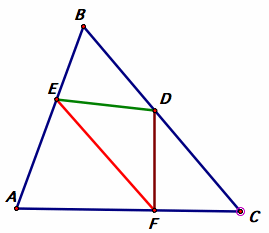A# Fagnano's problemSolution to Fagnano's problem.

Fagnano's problem is as follows: in a given acute triangle ABC, find the inscribed triangle whose perimeter is as small as possible. The answer is the orthic triangle of ABC, that is, the triangle (DEF) whose vertices are endpoints of the altitudes from each of the vertices of ABC. The problem was proposed and solved using calculus by Giovanni Fagnano (1715–1797) in 1775. Once the answer became known, several purely geometric solutions were also discovered.